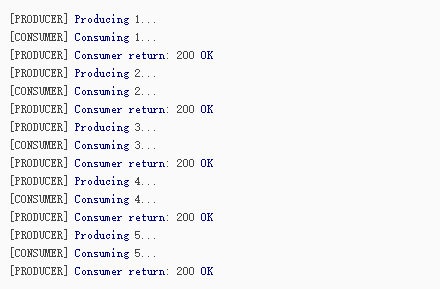# 一篇文章带你读懂Python的协程```def A():
print '1'
print '2'
print '3'
def B():
print 'x'
print 'y'
print 'z'```

```1
2
x
y
3
z```

Python对协程的支持还非常有限，用在generator中的yield可以一定程度上实现协程。虽然支持不完全，但已经可以发挥相当大的威力了。

```import timedef consumer():
r = ''
while True:
n = yield r        if not n:            return
print('[CONSUMER] Consuming %s...' % n)
time.sleep(1)
r = '200 OK'def produce(c):
c.next()
n = 0
while n < 5:
n = n + 1
print('[PRODUCER] Producing %s...' % n)
r = c.send(n)
print('[PRODUCER] Consumer return: %s' % r)
c.close()if __name__=='__main__':
c = consumer()
produce(c)```consumer通过yield拿到消息，处理，又通过yield把结果传回；

produce拿到consumer处理的结果，继续生产下一条消息；

produce决定不生产了，通过c.close()关闭consumer，整个过程结束。

“子程序就是协程的一种特例。”

Python开发开发项目